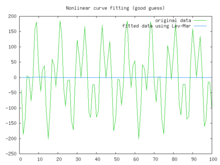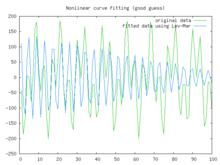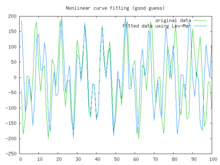# Levenberg–Marquardt algorithm

﻿
Levenberg–Marquardt algorithm

In mathematics and computing, the Levenberg–Marquardt algorithm (LMA) provides a numerical solution to the problem of minimizing a function, generally nonlinear, over a space of parameters of the function. These minimization problems arise especially in least squares curve fitting and nonlinear programming.

The LMA interpolates between the Gauss–Newton algorithm (GNA) and the method of gradient descent. The LMA is more robust than the GNA, which means that in many cases it finds a solution even if it starts very far off the final minimum. For well-behaved functions and reasonable starting parameters, the LMA tends to be a bit slower than the GNA. LMA can also be viewed as Gauss–Newton using a trust region approach.

The LMA is a very popular curve-fitting algorithm used in many software applications for solving generic curve-fitting problems. However, the LMA finds only a local minimum, not a global minimum.

## The problem

The primary application of the Levenberg–Marquardt algorithm is in the least squares curve fitting problem: given a set of m empirical datum pairs of independent and dependent variables, (xi, yi), optimize the parameters β of the model curve f(x,β) so that the sum of the squares of the deviations$S(\boldsymbol \beta) = \sum_{i=1}^m [y_i - f(x_i, \ \boldsymbol \beta) ]^2$

becomes minimal.

## The solution

Like other numeric minimization algorithms, the Levenberg–Marquardt algorithm is an iterative procedure. To start a minimization, the user has to provide an initial guess for the parameter vector, β. In cases with only one minimum, an uninformed standard guess like βT=(1,1,...,1) will work fine; in cases with multiple minima, the algorithm converges only if the initial guess is already somewhat close to the final solution.

In each iteration step, the parameter vector, β, is replaced by a new estimate, β + δ. To determine δ, the functions$f(x_i,\boldsymbol \beta+\boldsymbol \delta)$ are approximated by their linearizations$f(x_i,\boldsymbol \beta+\boldsymbol \delta) \approx f(x _i,\boldsymbol \beta) + J_i \boldsymbol \delta \!$

where$J_i=\frac{\partial f(x_i,\boldsymbol\beta)}{\partial \boldsymbol\beta}$

is the gradient (row-vector in this case) of f with respect to β.

At its minimum, the sum of squares, S(β), the gradient of S with respect to δ will be zero. The above first-order approximation of$f(x_i,\boldsymbol \beta+\boldsymbol \delta)$ gives$S(\boldsymbol\beta+\boldsymbol\delta) \approx \sum_{i=1}^m \left( y_i - f(x_i,\boldsymbol\beta) - J_i \boldsymbol\delta\right)^2$.

Or in vector notation,$S(\boldsymbol\beta+\boldsymbol\delta) \approx \|\mathbf{y} - \mathbf{f}(\boldsymbol\beta) - \mathbf{J}\boldsymbol\delta\|^2$.

Taking the derivative with respect to δ and setting the result to zero gives:$\mathbf{(J^{T}J)\boldsymbol \delta = J^{T} [y - f(\boldsymbol \beta)]} \!$

where$\mathbf{J}$ is the Jacobian matrix whose ith row equals Ji, and where$\mathbf{f}$ and$\mathbf{y}$ are vectors with ith component$f(x_i,\boldsymbol \beta)$ and yi, respectively. This is a set of linear equations which can be solved for δ.

Levenberg's contribution is to replace this equation by a "damped version",$\mathbf{(J^{T}J + \lambda I)\boldsymbol \delta = J^{T} [y - f(\boldsymbol \beta)]}\!$

where I is the identity matrix, giving as the increment, δ, to the estimated parameter vector, β.

The (non-negative) damping factor, λ, is adjusted at each iteration. If reduction of S is rapid, a smaller value can be used, bringing the algorithm closer to the Gauss–Newton algorithm, whereas if an iteration gives insufficient reduction in the residual, λ can be increased, giving a step closer to the gradient descent direction. Note that the gradient of S with respect to β equals$-2(\mathbf{J}^{T} [\mathbf{y} - \mathbf{f}(\boldsymbol \beta) ] )^T$. Therefore, for large values of λ, the step will be taken approximately in the direction of the gradient. If either the length of the calculated step, δ, or the reduction of sum of squares from the latest parameter vector, β + δ, fall below predefined limits, iteration stops and the last parameter vector, β, is considered to be the solution.

Levenberg's algorithm has the disadvantage that if the value of damping factor, λ, is large, inverting JTJ + λI is not used at all. Marquardt provided the insight that we can scale each component of the gradient according to the curvature so that there is larger movement along the directions where the gradient is smaller. This avoids slow convergence in the direction of small gradient. Therefore, Marquardt replaced the identity matrix, I, with the diagonal matrix consisting of the diagonal elements of JTJ, resulting in the Levenberg–Marquardt algorithm:$\mathbf{(J^T J + \lambda\, diag(J^T J))\boldsymbol \delta = J^T [y - f(\boldsymbol \beta)]}\!$.

A similar damping factor appears in Tikhonov regularization, which is used to solve linear ill-posed problems, as well as in ridge regression, an estimation technique in statistics.

### Choice of damping parameter

Various more-or-less heuristic arguments have been put forward for the best choice for the damping parameter λ. Theoretical arguments exist showing why some of these choices guaranteed local convergence of the algorithm; however these choices can make the global convergence of the algorithm suffer from the undesirable properties of steepest-descent, in particular very slow convergence close to the optimum.

The absolute values of any choice depends on how well-scaled the initial problem is. Marquardt recommended starting with a value λ0 and a factor ν>1. Initially setting λ=λ0 and computing the residual sum of squares S(β) after one step from the starting point with the damping factor of λ=λ0 and secondly with λ0/ν. If both of these are worse than the initial point then the damping is increased by successive multiplication by ν until a better point is found with a new damping factor of λ0νk for some k.

If use of the damping factor λ/ν results in a reduction in squared residual then this is taken as the new value of λ (and the new optimum location is taken as that obtained with this damping factor) and the process continues; if using λ/ν resulted in a worse residual, but using λ resulted in a better residual then λ is left unchanged and the new optimum is taken as the value obtained with λ as damping factor.

## ExamplePoor fitBetter fitBest fit

In this example we try to fit the function y = acos(bX) + bsin(aX) using the Levenberg–Marquardt algorithm implemented in GNU Octave as the leasqr function. The 3 graphs Fig 1,2,3 show progressively better fitting for the parameters a=100, b=102 used in the initial curve. Only when the parameters in Fig 3 are chosen closest to the original, are the curves fitting exactly. This equation is an example of very sensitive initial conditions for the Levenberg–Marquardt algorithm. One reason for this sensitivity is the existence of multiple minima — the function cos(βx) has minima at parameter value$\hat \beta$ and$\hat \beta +2n \pi.$

Wikimedia Foundation. 2010.

### Look at other dictionaries:

• Levenberg-Marquardt-Algorithmus — Der Levenberg Marquardt Algorithmus, benannt nach Kenneth Levenberg und Donald Marquardt, ist ein numerischer Optimierungsalgorithmus zur Lösung nichtlinearer Ausgleichs Probleme mit Hilfe der Methode der kleinsten Quadrate. Das Verfahren… …   Deutsch Wikipedia

• Levenberg-Marquardt — Algorithme de Levenberg Marquardt L’algorithme de Levenberg Marquardt, ou algorithme LM, permet d obtenir une solution numérique au problème de minimisation d une fonction, souvent non linéaire et dépendant de plusieurs variables. L algorithme… …   Wikipédia en Français

• Algorithme de Levenberg-Marquardt — L’algorithme de Levenberg Marquardt, ou algorithme LM, permet d obtenir une solution numérique au problème de minimisation d une fonction, souvent non linéaire et dépendant de plusieurs variables. L algorithme interpole l algorithme de Gauss… …   Wikipédia en Français

• Algorithme De Levenberg-Marquardt — L’algorithme de Levenberg Marquardt, ou algorithme LM, permet d obtenir une solution numérique au problème de minimisation d une fonction, souvent non linéaire et dépendant de plusieurs variables. L algorithme interpole l algorithme de Gauss… …   Wikipédia en Français

• Algorithme de levenberg-marquardt — L’algorithme de Levenberg Marquardt, ou algorithme LM, permet d obtenir une solution numérique au problème de minimisation d une fonction, souvent non linéaire et dépendant de plusieurs variables. L algorithme interpole l algorithme de Gauss… …   Wikipédia en Français

• Marquardt-Levenberg — Algorithme de Levenberg Marquardt L’algorithme de Levenberg Marquardt, ou algorithme LM, permet d obtenir une solution numérique au problème de minimisation d une fonction, souvent non linéaire et dépendant de plusieurs variables. L algorithme… …   Wikipédia en Français

• Gauss–Newton algorithm — The Gauss–Newton algorithm is a method used to solve non linear least squares problems. It can be seen as a modification of Newton s method for finding a minimum of a function. Unlike Newton s method, the Gauss–Newton algorithm can only be used… …   Wikipedia

• Donald Marquardt — Donald W. Marquardt (March 13, 1929 – July 5, 1997) was an American statistician, the rediscoverer of the Levenberg–Marquardt nonlinear least squares fitting algorithm. Marquardt joined DuPont in 1953 and worked there for 39 years. He also… …   Wikipedia

• Donald Marquardt — Donald W. Marquardt (13 mars 1929 5 juillet 1997) est un statisticien américain qui a publié l algorithme d ajustement aux moindres carrés d équations non linéaires, développé par Kenneth Levenberg et connu sous le nom d algorithme de… …   Wikipédia en Français

• Criss-cross algorithm — This article is about an algorithm for mathematical optimization. For the naming of chemicals, see crisscross method. The criss cross algorithm visits all 8 corners of the Klee–Minty cube in the worst case. It visits 3 additional… …   Wikipedia